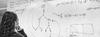# 1st Semester 2023/24: On the Equivalence of Scientific Theories

Instructors
Sebastian De Haro
Description

In the recent philosophy of science literature, there is an ongoing discussion about what are appropriate criteria for theoretical equivalence, i.e. full equivalence between scientific theories. Among the proposed criteria, categorical equivalence and duality-based criteria stand out as candidates that can be tested on actual scientific theories.

However, categorical equivalence has been criticized for being too "liberal", i.e. to logically weak, because it counts as equivalent theories that one would not necessarily count as equivalent. (For example, it counts as equivalent a formulation of Mawell's theory of electromagnetism based on a gauge potential, and one based on the gauge orbits of the gauge potential: and these formulations have been argued to be,at least in some important situations, inequivalent.)

On the other hand, dualities (understood as appropriate isomorphisms between physical theories) have so far passed all the tests, in the sense that they enter in correctly into verdicts of theoretical equivalence, in a large class of exampes that have been studied in physics.

This project aims to compare the duality-based criterion of equivalence with categorical equivalence. Specifically, the aim is to study possible reformulations of dualities in terms of categorical equivalence. By doing so, one hopes to, first, give dualities a more rigorous mathematical formulation and, second, understand more precisely how duality and categorical equivalence differ, i.e. why categorical equivalence is too weak, while dualities have the right strength.

If time permits, a recent reformulation of dualities will be studied, which can be used for scientific theories that are syntactically formulated, and which can help compare dualities to other syntactic of equivalence.

Organisation

ILLC, UvA

Prerequisites

The project has several components (category theory, isomorphisms between scientific theories, and syntactic formulations of scientific theories), but it does not require knowledge of each of them. Rather, this diversity of components will allow the student to focus on a specific aspect of the research, depending on their prior knowledge.

Assessment

Paper.

References

James Weatherall (2019), 'Categorical Equivalence in Physics', Part 1 and Part 2.

Sebastian De Haro and Jeremy Butterfield (2024), The Philosophy and Physics of Duality, forthcoming at Oxford University Press. The relevant chapters are available on request.

Hans Halvorson (202), 'What Scientific Theories Could Not Be'.